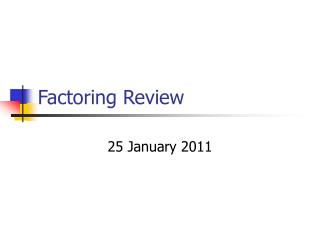DownloadDownload PresentationFactoring Review

# Factoring Review

Download Presentation## Factoring Review

- - - - - - - - - - - - - - - - - - - - - - - - - - - E N D - - - - - - - - - - - - - - - - - - - - - - - - - - -
##### Presentation Transcript

1. Factoring Review 25 January 2011

2. Factoring • The process of rewriting an equation or expression as the product of its factors • Example: x2 + 3x + 2 = (x + 2)(x + 1) • Most common form is the quadratic form: ax2 + bx + c, a ≠ 0

3. Factoring (when a = 1) ax2 + bx + c = (x + ___ ) (x + ___ ) multiply to equal c and add up to equal b You can always check your answer by FOIL-ing!

4. Finding Factors of C • Identify the value of c • On your calculator, go to the y= screen • Type C/X into y1 • Go to the table • Any whole numbers (positive, non-decimal numbers) in the y1 column are factors of c

5. Example

6. Example #1

7. Example #2

8. Example #3

9. Your Turn: • Complete problems 1 – 4 on the “Factoring Practice” handout • Check your answer by FOIL-ing!

10. Difference of Squares • When we use it: • Usually in the form ax2 – c • Both a and c are perfect squares (the square root of each number is a whole number)

11. Example #1

12. Example #2

13. Your Turn: • Complete problems 5 – 10 on the “Factoring Practice” handout • Remember to check your answer by FOIL-ing!

14. Factoring (when a ≠ 1):The Welsh Method Pt. I • Multiply c and a • Rewrite the expression with the new value for c • Write (ax + )(ax + ) • Finish “factoring” the new expression • Reduce each set of parentheses by any common factors

15. Example #1

16. Example #2

17. Example #3

18. Your Turn: • Complete problems 11 – 20 on the “Factoring Practice” handout • Don’t forget to check by FOIL-ing!

19. GCF (Greatest Common Factor) • When we use it: when the all the terms share 1 or more factors • Factoring out GCFs save us time!!!

20. GCF (Greatest Common Factor) • Steps: • Identify any common factor(s) (including the GCF) • Factor out the common factor(s) • Factor the remaining expression if possible

21. Example #1

22. Example #2

23. Example #3

24. Your Turn: • Complete problems 1 – 10 on “Functions Practice Pt. II” handout

25. GCFs and The Welsh Method Make sure you factor out any GCFs or the Welsh Method doesn’t work!!!

26. Your Turn: • Complete problems 11 – 22 on the “Factoring Practice Part II” handout